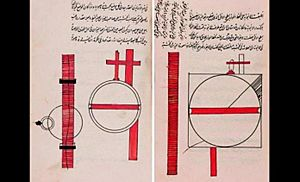# Al-Karaji facts for kids

Kids Encyclopedia Facts
Quick facts for kids
Abū Bakr al-KarajīDiagrams from Al-Karaji's work on "hidden waters"
Born 953
Died 1029 (aged 75–76)
Nationality Persian
Main interests
Mathematics, Engineering

Abū Bakr Muḥammad ibn al Ḥasan al-Karajī (Persian: ابو بکر محمد بن الحسن الکرجی; c. 953 – c. 1029) was a 10th-century Persian mathematician and engineer who flourished at Baghdad. He was born in Karaj, a city near Tehran. His three principal surviving works are mathematical: Al-Badi' fi'l-hisab (Wonderful on calculation), Al-Fakhri fi'l-jabr wa'l-muqabala (Glorious on algebra), and Al-Kafi fi'l-hisab (Sufficient on calculation).

## Work

Al-Karaji wrote on mathematics and engineering. Some consider him to be merely reworking the ideas of others (he was influenced by Diophantus) but most regard him as more original, in particular for the beginnings of freeing algebra from geometry. Among historians, his most widely studied work is his algebra book al-fakhri fi al-jabr wa al-muqabala, which survives from the medieval era in at least four copies.

In his book "Extraction of hidden waters" he has mentioned that earth is spherical in shape but considers it the centre of the universe long before Galileo Galilei, Johannes Kepler or Isaac Newton, but long after Aristotle and Ptolemy. He expounded the basic principles of hydrology and this book reveals a profound knowledge of this science and has been described as the oldest extant text in this field.

He systematically studied the algebra of exponents, and was the first to realise that the sequence x, x^2, x^3,... could be extended indefinitely; and the reciprocals 1/x, 1/x^2, 1/x^3,... . However, since for example the product of a square and a cube would be expressed, in words rather than in numbers, as a square-cube, the numerical property of adding exponents was not clear.

His work on algebra and polynomials gave the rules for arithmetic operations for adding, subtracting and multiplying polynomials; though he was restricted to dividing polynomials by monomials.

F. Woepcke was the first historian to realise the importance of al-Karaji's work and later historians mostly agree with his interpretation. He praised Al-Karaji for being the first who introduced the theory of algebraic calculus.

Al-Karaji gave the first formulation of the binomial coefficients and the first description of Pascal's triangle. He is also credited with the discovery of the binomial theorem.

In a now lost work known only from subsequent quotation by al-Samaw'al Al-Karaji introduced the idea of argument by mathematical induction. As Katz says

Another important idea introduced by al-Karaji and continued by al-Samaw'al and others was that of an inductive argument for dealing with certain arithmetic sequences. Thus al-Karaji used such an argument to prove the result on the sums of integral cubes already known to Aryabhata [...] Al-Karaji did not, however, state a general result for arbitrary n. He stated his theorem for the particular integer 10 [...] His proof, nevertheless, was clearly designed to be extendable to any other integer. [...] Al-Karaji's argument includes in essence the two basic components of a modern argument by induction, namely the truth of the statement for n = 1 (1 = 13) and the deriving of the truth for n = k from that of n = k - 1. Of course, this second component is not explicit since, in some sense, al-Karaji's argument is in reverse; this is, he starts from n = 10 and goes down to 1 rather than proceeding upward. Nevertheless, his argument in al-Fakhri is the earliest extant proof of the sum formula for integral cubes.In Spanish: Al-Karaŷí para niños